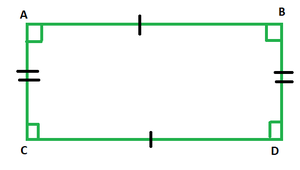Open in App
Not now

# If the length and breadth of a rectangle are 3.2 m and 150 cm, then find the area

• Last Updated : 20 Nov, 2021

Everything that surrounds us has a definite shape except for liquids. Starting from our mobile phones, laptops to our cupboards, water bottles, microwave oven, kitchen bowls, water tanks, LPG pipelines, etc. all have a definite shape. Clearly, some of these objects are used to store stuff for us. Such shapes are categorized on certain criteria in Mathematics and studied to either create more shapes like them or to improvise the existing ones by either covering them or decorating them. Furthermore, their storage capacity is also worked upon in mathematics to use them to hold things.

### What is Mensuration?

Mensuration is that branch of mathematics that deals with the computation of dimensions and dimensional quantities pertaining to various geometrical shapes. It is the act of measuring, be its length, breadth, height, area, or any such parameter pertinent to all geometrical shapes we see around us and use everyday.

Basic Terminology

• Two-Dimensional Shape: As the name suggests, such shapes have only two proportions: length and breadth. Rectangle, square, triangle fall under this category.
• Three-Dimensional Shape: Those shapes which have three proportions/ measurements are called three-dimensional shapes. Such proportions are length, breadth, and height. Cube, Cuboid fall under this category of shapes.
• Area: Such a parameter that determines the region occupied by a shape. It can only be calculated for two- dimensional shapes.
• Perimeter: Adding up the lengths of sides of a 2- D shape yields its perimeter. It is used to depict the length of an object around its sides.

Such a geometrical shape that is formed by joining four points in such a way that at least three of them do not lie in the same straight line is called a quadrilateral. If the points happen to be in the same line, then we would get a ray or a line segment instead of an enclosed shape. Quadrilaterals, as the name suggests, have 4 sides, angles, edges, and vertices since the word ‘quad’ means four. The sum of all the four angles of a quadrilateral must always be 360 degrees. Quadrilaterals are assigned different names on the basis of lengths of their sides as either square, rhombus, rectangle, parallelogram, trapezium, etc.

Rectangle

A quadrilateral whose all angles measure 90 degrees and whose parallel sides are equal is called a rectangle. It is a two-dimensional shape that has only two measurements pertaining to it, i.e., length and breadth. The figure below depicts a rectangle ABCD, whose angles are equal to 90 degrees each and the parallel sides are equal in length.The sides AB and CD represent the length of the rectangle and AC and BD represent the breadth. Like any other quadrilateral, the sum of interior angles of rectangle ABCD is 360 degrees.

Area of a Rectangle

The area of a rectangle is computed by multiplying both its dimensions, keeping in mind they both are of the same unit. Thus, in the figure above, if the area of rectangle ABCD were to be calculated, it would come out to be (AB × CD) sq. units.

Thus, area of rectangle = l × b sq. units.

### If the length and breadth of a rectangle are 3.2 m and 150 cm, then find the area.

Solution:

Given: Length = 3.2 m and breadth = 150 cm = 1.5 m

Area = L × B

= (3.2 × 1.5) m2

Area of the rectangle = 4.8 m2

### Similar Problems

Question 1. Find the area of a rectangle whose length and breadth of a rectangle are 1.2 m and 150 cm.

Solution:

Given: Length = 1.2 m and breadth = 150 cm = 1.5 m

Area = L × B

= (1.2 × 1.5) m2

Area of the rectangle = 1.8 m2

Question 2. Find the area of a rectangle whose length and breadth of a rectangle are 5 m and 25 cm.

Solution:

Given: Length = 5 m and breadth = 25 cm = 0.25 m

Area = L × B

= (5 × 0.25) m2

Area of the rectangle = 1.25 m2

Question 3. Find the area of a rectangle whose length and breadth of a rectangle are 4.5 m and 450 cm.

Solution:

Given: Length = 4.5 m and breadth = 450 cm = 4.5 m

Area = L × B

= (4.5 × 4.5) m2

Area of the rectangle = 20.25 m2

My Personal Notes arrow_drop_up